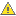﻿ Trees
Nevron .NET Vision Documentation
Trees

A tree graph (or simply tree) is a connected directed acyclic graph, in which each vertex has only one incoming edge except the root vertex, for which there is no incoming edge. A tree is represented by an instance of NTree class (derived from NGraphPartContainer class). The vertices of a tree are instances of the NTreeVertex class. The edges of a tree are instances of the NTreeEdge class.

Creating a Tree

A tree graph representing the connected structure of shapes can be constructed with the help of the NGraphBuilder and NShapeGraphAdapter classes. The following example creates a tree, which represents the connected component in which a shape resides:

C#
Copy Code
```NGraphBuilder graphBuilder = new NGraphBuilder(new NShapeGraphAdapter(), new NGraphPartFactory());
NObjectGraphPartMap map;
NTree tree = graphBuilder.BuildTree(shape, out map);
```
Visual Basic
Copy Code
```Dim graphBuilder As New NGraphBuilder(New NShapeGraphAdapter(), New NGraphPartFactory())
Dim map As NObjectGraphPartMap
Dim tree As NTree = graphBuilder.BuildTree(shape, map)
```

The output NObjectGraphPartMap instance is a bi-map of (object - to/from - graph part), which means that you can easily get the graph part, which represents a shape and vice versa:

C#
Copy Code
```// get the graph part which represents a shape
NGraphPart part = map.GetPartFromObject(shape);
// get the shape which the part represents
NShape shape = (NShape)map.GetObjectFromPart(part);
```
Visual Basic
Copy Code
```' get the graph part which represents a shape
Dim part NGraphPart = map.GetPartFromObject(shape)
' get the shape which the part represents
Dim shape NShape = map.GetObjectFromPart(part)
```The graph builder will create a tree rooted at the specified shape, which represents the depth first spanning tree of a directed graph, with the shape as start vertex. Alternatively you can obtain a spanning tree of a graph. See Graphs for more information.

The common tree navigation routines are exposed by the methods and properties of the NTreeVertex and NTreeEdge classes. For example:

C#
Copy Code
```// get the child vertex of an edge
NTreeVertex childVertex = edge.Child;

// get the parent vertex of an edge
NTreeVertex parentVertex = edge.Parent;

// get the children of a vertex
NTreeVertexList children = vertex.Children;

// get the parent of a vertex
NTreeVertex parent = vertex.Parent;
```
Visual Basic
Copy Code
```' get the child vertex of an edge
Dim childVertex As NTreeVertex = edge.Child

' get the parent vertex of an edge
Dim parentVertex As NTreeVertex = edge.Parent

' get the children of a vertex
Dim children As NTreeVertexList = vertex.Children

' get the parent of a vertex
Dim parent As NTreeVertex = vertex.Parent
```
Tree Checks
The  NTree class exposes several properties, which can help you retrieve useful information about it. Currently these are: IsBinary.
Tree Traversal

The NTree class has built-in support for the following types of tree traversal methods:

• Post Order Traversal - in this type of tree traversal the algorithm first visits the children and then visits the parent. It is implemented by the PostOrderTraversal method.
• Pre Order Traversal - in this type of tree traversal the algorithm first visits the parent and then visits the children. It is implemented by the PreOrderTraversal method.
• Breadth First Traversal - in this type of tree traversal the algorithm visits the vertices by levels. It is implemented by the BreadthFirstTraversal method.

All types of tree traversal methods use the visitor design-pattern, which means that they accept an instance of the NGraphPartVisitor class. When a vertex or an edge has been visited the algorithm will call the Visit method of the visitor, passing as argument the part which has been visited.